# 吴恩达Coursera深度学习课程 DeepLearning.ai 提炼笔记（2-1）-- 深度学习的实践方面

GitHubhttps://github.com/KoalaTree
2017 年 09 月 28 日

## 改善深层神经网络：超参数调试、正则化以及优化 —深度学习的实践方面

### 1. 训练、验证、测试集

• 训练集（train set）：用训练集对算法或模型进行训练过程；

• 验证集（development set）：利用验证集或者又称为简单交叉验证集（hold-out cross validation set）进行交叉验证，选择出最好的模型；

• 测试集（test set）：最后利用测试集对模型进行测试，获取模型运行的无偏估计。

#### 小数据时代

• 无验证集的情况：70% / 30%；
• 有验证集的情况：60% / 20% / 20%；

#### 大数据时代

• 100万数据量：98% / 1% / 1%；
• 超百万数据量：99.5% / 0.25% / 0.25%（或者99.5% / 0.4% / 0.1%）

#### Notation

• 建议验证集要和训练集来自于同一个分布，可以使得机器学习算法变得更快；
• 如果不需要用无偏估计来评估模型的性能，则可以不需要测试集。

### 2. 偏差、方差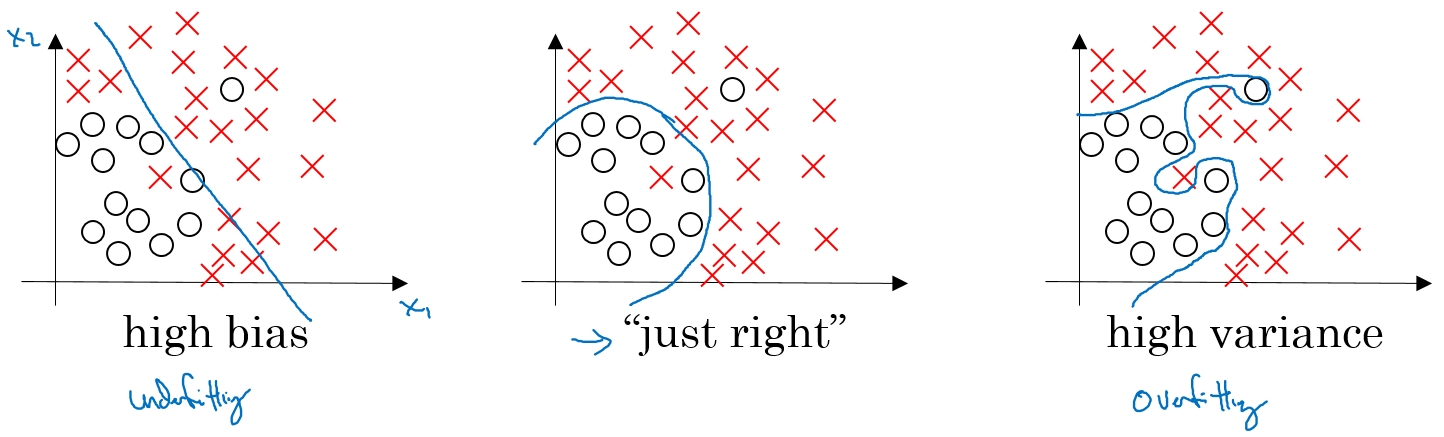#### 例子：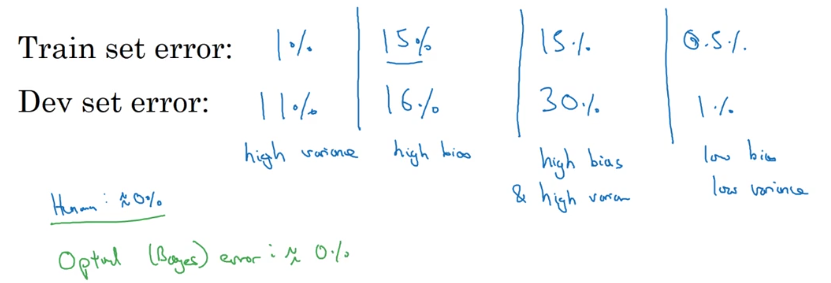High bias and high variance的情况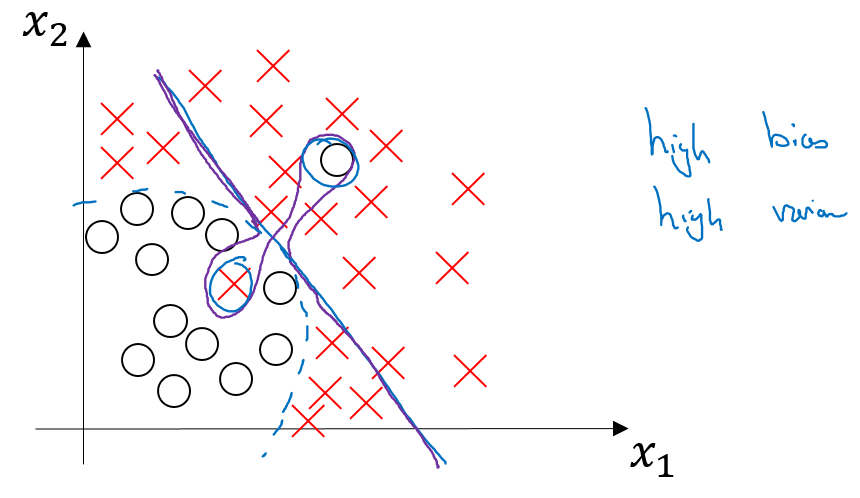### 3. 机器学习的基本方法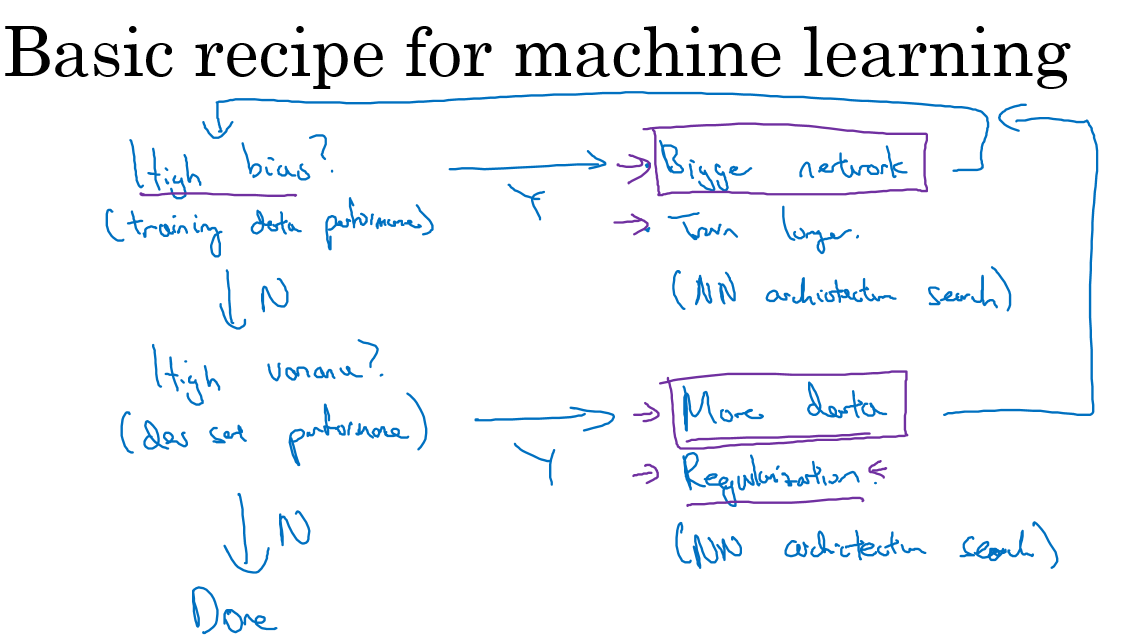• 1.是否存在High bias ?
• 增加网络结构，如增加隐藏层数目；
• 训练更长时间；
• 寻找合适的网络架构，使用更大的NN结构；
• 2.是否存在High variance
• 获取更多的数据；
• 正则化（ regularization）；
• 寻找合适的网络结构；

### 4. 正则化（regularization）

#### Logistic regression

J(w,b)=1mi=1ml(y^(i),y(i))+λ2m||w||22

• L2正则化：λ2m||w||22=λ2mj=1nxw2j=λ2mwTw$\dfrac{\lambda}{2m}||w||_{2}^{2} = \dfrac{\lambda}{2m}\sum\limits_{j=1}^{n_{x}} w_{j}^{2}=\dfrac{\lambda}{2m}w^{T}w$
• L1正则化：λ2m||w||1=λ2mj=1nx|wj|$\dfrac{\lambda}{2m}||w||_{1}=\dfrac{\lambda}{2m}\sum\limits_{j=1}^{n_{x}}|w_{j}|$

#### Neural network

J(w,b,,w[L],b[L])=1mi=1ml(y^(i),y(i))+λ2ml=1L||w[l]||2F

Weight decay

dW[l]=(form_backprop)+λmW[l]

W[l]:=W[l]αdW[l]

W[l]:=W[l]α[(form_backprop)+λmW[l]]=W[l]αλmW[l]α(form_backprop)=(1αλm)W[l]α(form_backprop)

### 5. 为什么正则化可以减小过拟合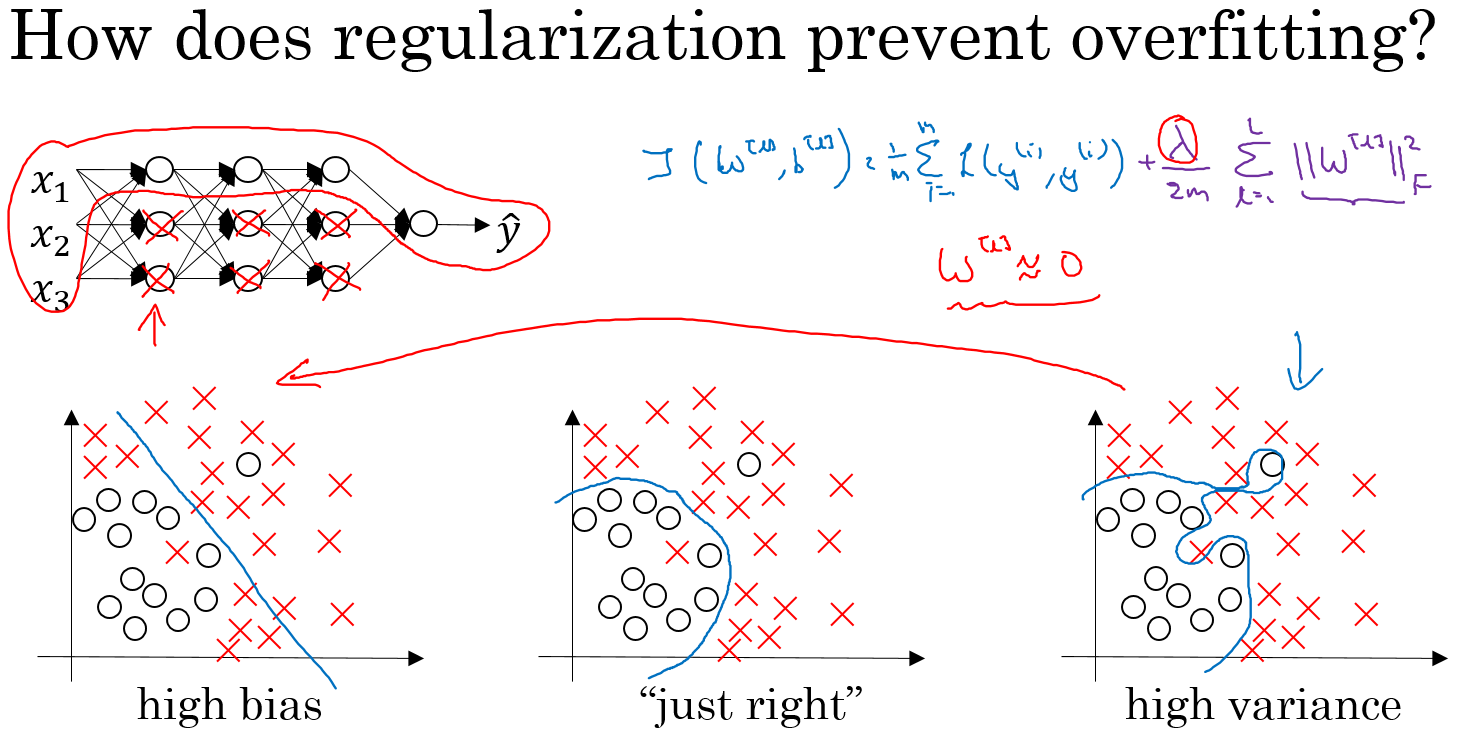J(w,b,,w[L],b[L])=1mi=1ml(y^(i),y(i))+λ2ml=1L||w[l]||2F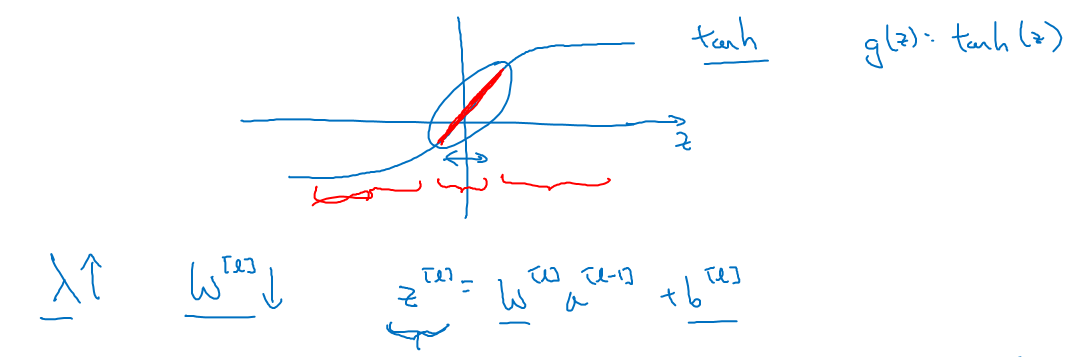λ$\lambda$增大，导致W[l]$W^{[l]}$减小，Z[l]=W[l]a[l1]+b[l]$Z^{[l]}=W^{[l]}a^{[l-1]}+b^{[l]}$便会减小，由上图可知，在z$z$较小的区域里，tanh(z)$\tanh(z)$函数近似线性，所以每层的函数就近似线性函数，整个网络就成为一个简单的近似线性的网络，从而不会发生过拟合。

### 6. Dropout 正则化

Dropout（随机失活）就是在神经网络的Dropout层，为每个神经元结点设置一个随机消除的概率，对于保留下来的神经元，我们得到一个节点较少，规模较小的网络进行训练。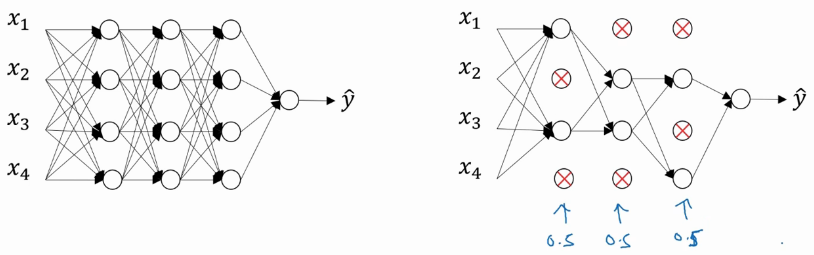#### 实现Dropout的方法：反向随机失活（Inverted dropout）

keep_prob = 0.8  # 设置神经元保留概率
d3 = np.random.rand(a3.shape, a3.shape) < keep_prob
a3 = np.multiply(a3, d3)
a3 /= keep_prob

Inverted dropout通过对“a3 /= keep_prob”,则保证无论keep_prob设置为多少，都不会对Z$Z^{}$的期望值产生影响。

Notation：在测试阶段不要用dropout，因为那样会使得预测结果变得随机。

### 7. 理解 Dropout

=1.0，神经元多的层，则会将keep_prob设置的较小。

dropout的一大缺点就是其使得 Cost function不能再被明确的定义，以为每次迭代都会随机消除一些神经元结点，所以我们无法绘制出每次迭代J(W,b)$J(W,b)$下降的图，如下：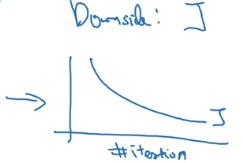• 关闭dropout功能，即设置 keep_prob = 1.0；
• 运行代码，确保J(Wb)$J(W，b)$函数单调递减；
• 再打开dropout函数。

### 8. 其他正则化方法

• 数据扩增（Data augmentation）：通过图片的一些变换，得到更多的训练集和验证集；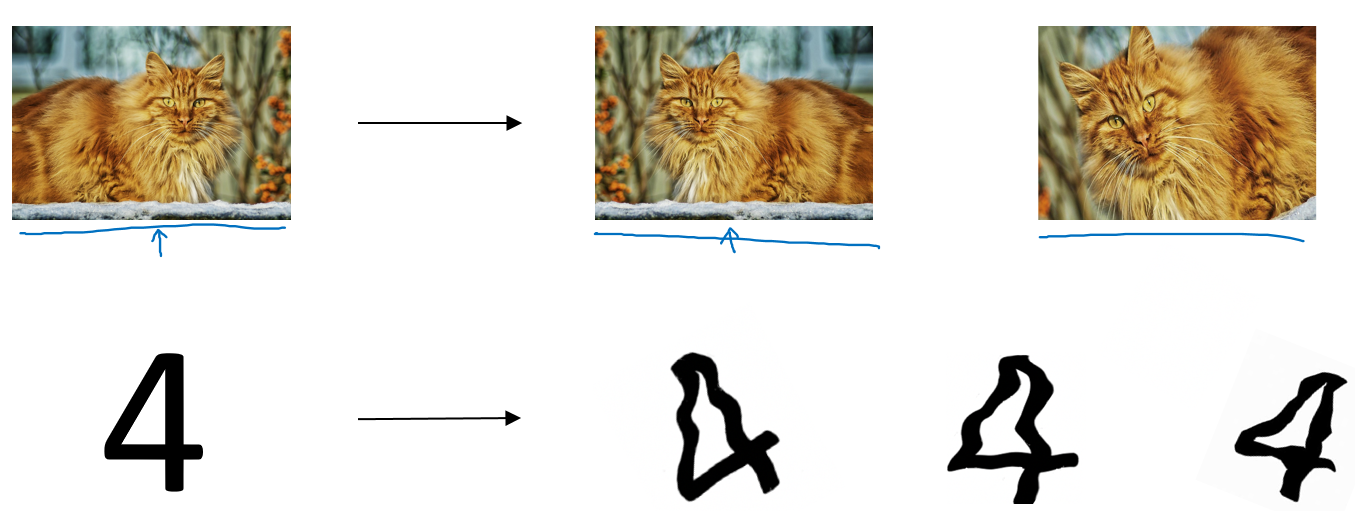• Early stopping：在交叉验证集的误差上升之前的点停止迭代，避免过拟合。这种方法的缺点是无法同时解决bias和variance之间的最优。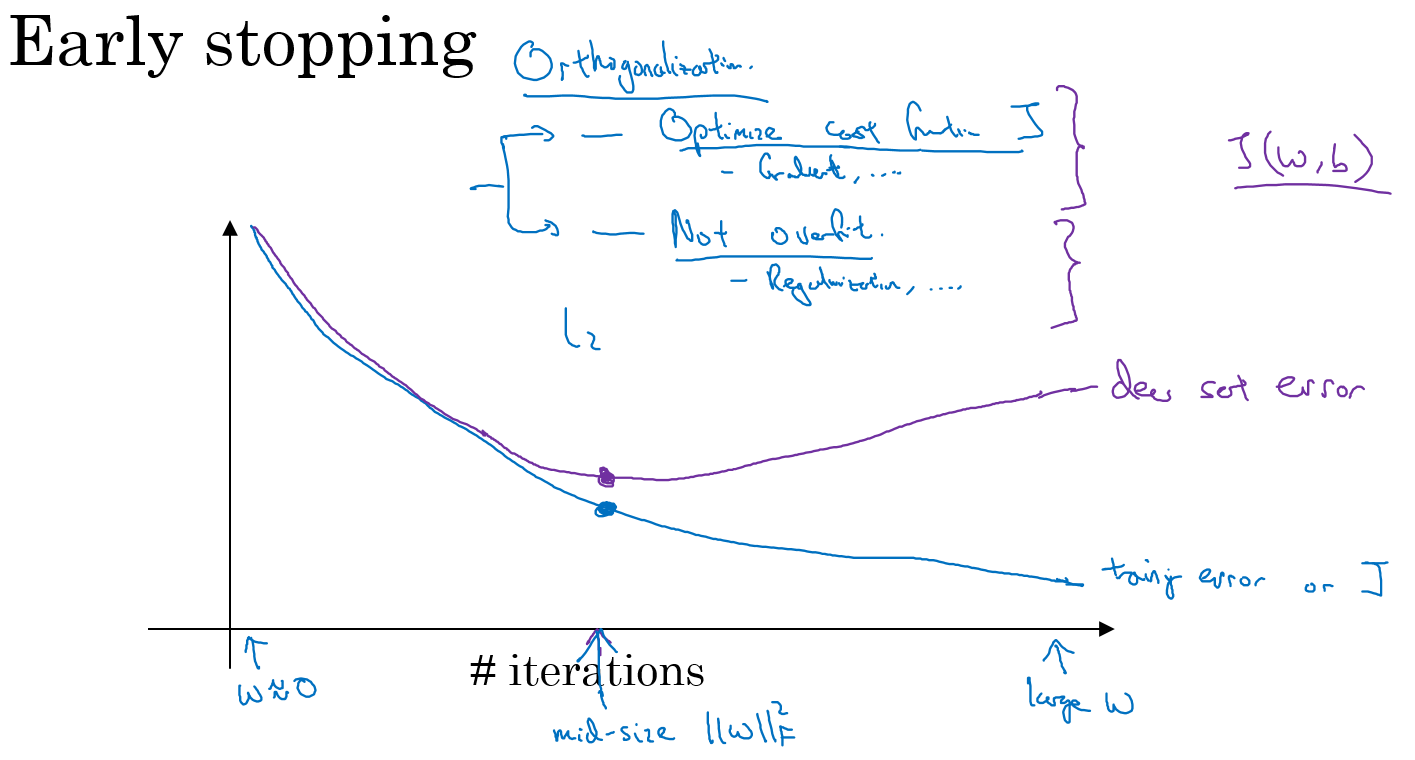### 9. 归一化输入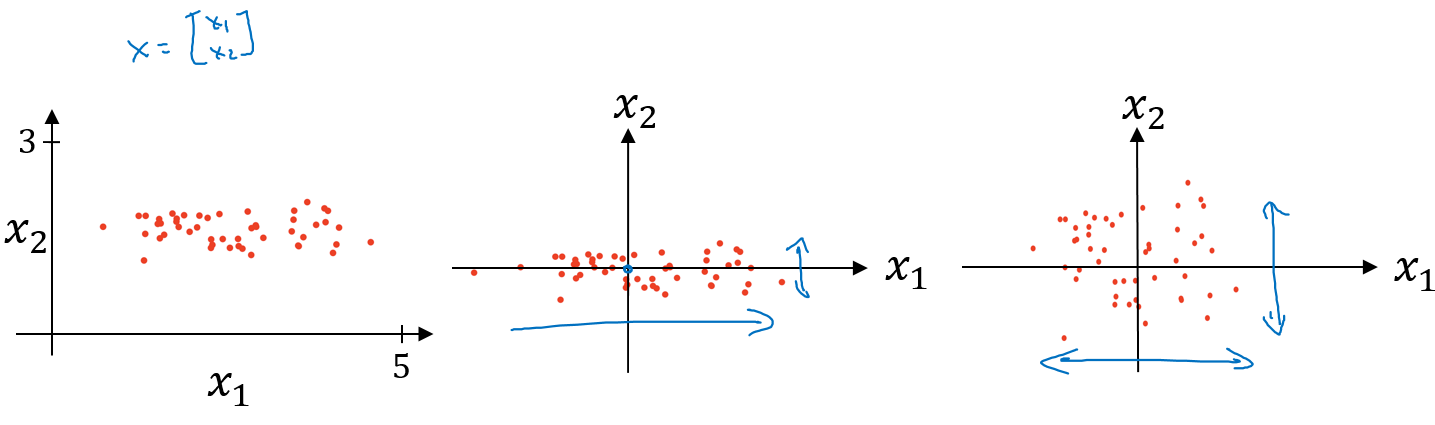• 计算每个特征所有样本数据的均值：μ=1mi=1mx(i)$\mu = \dfrac{1}{m}\sum\limits_{i=1}^{m}x^{(i)}$
• 减去均值得到对称的分布：x:=xμ$x : =x-\mu$
• 归一化方差：σ2=1mi=1mx(i)2$\sigma^{2} = \dfrac{1}{m}\sum\limits_{i=1}^{m}x^{(i)^{2}}$x=x/σ2$x = x/\sigma^{2}$

#### 使用归一化的原因：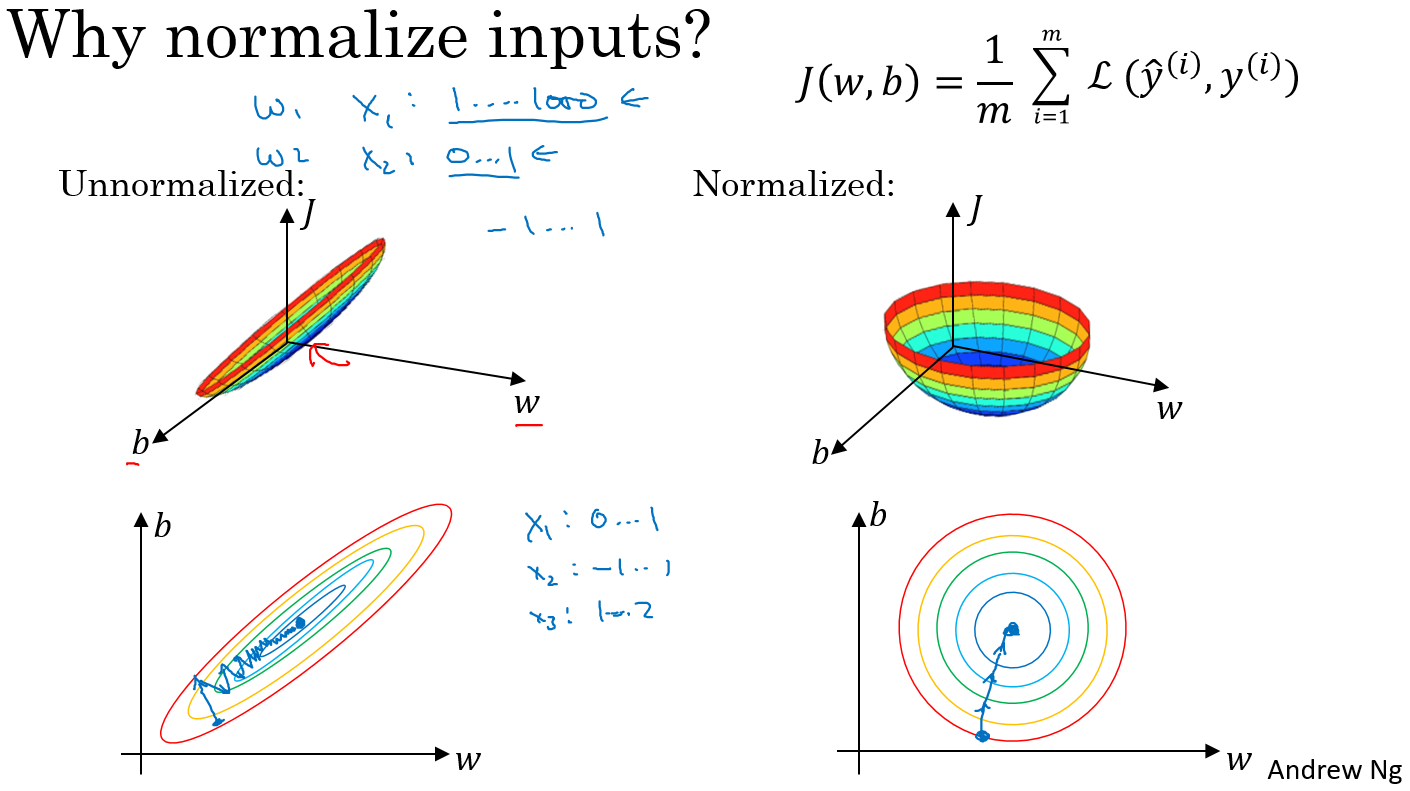### 10. 梯度消失与梯度爆炸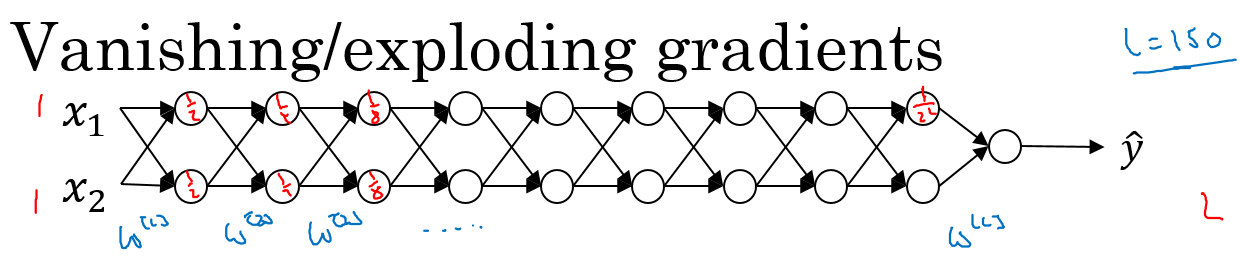y^=W[L]W[L1]WWX

• W[l]$W^{[l]}$的值大于1的情况：
如：W[l]=[1.5001.5]$W^{[l]}=\left[ \begin{array}{l} 1.5 & 0 \\ 0 & 1.5\end{array} \right]$，那么最终，y^=W[L][1.5 001.5]L1X$\hat y = W^{[L]}\left[ \begin{array}{l} 1.5 & 0 \\\ 0 & 1.5\end{array} \right]^{L-1}X$，激活函数的值将以指数级递增；
• W[l]$W^{[l]}$的值小于1的情况：
如：W[l]=[0.5000.5]$W^{[l]}=\left[ \begin{array}{l} 0.5 & 0 \\ 0 & 0.5\end{array} \right]$，那么最终，y^=W[L][0.5 000.5]L1X$\hat y = W^{[L]}\left[ \begin{array}{l} 0.5 & 0 \\\ 0 & 0.5\end{array} \right]^{L-1}X$，激活函数的值将以指数级递减。

### 11. 利用初始化缓解梯度消失和爆炸问题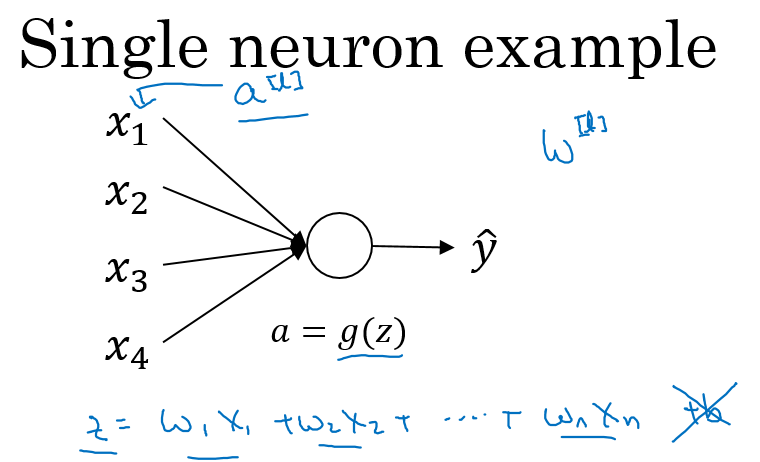WL = np.random.randn(WL.shape,WL.shape)* np.sqrt(1/n)

• 激活函数使用Relu：Var(wi)=2n$Var(w_{i})=\dfrac{2}{n}$
• 激活函数使用tanh：Var(wi)=1n$Var(w_{i})=\dfrac{1}{n}$

### 12. 梯度的数值逼近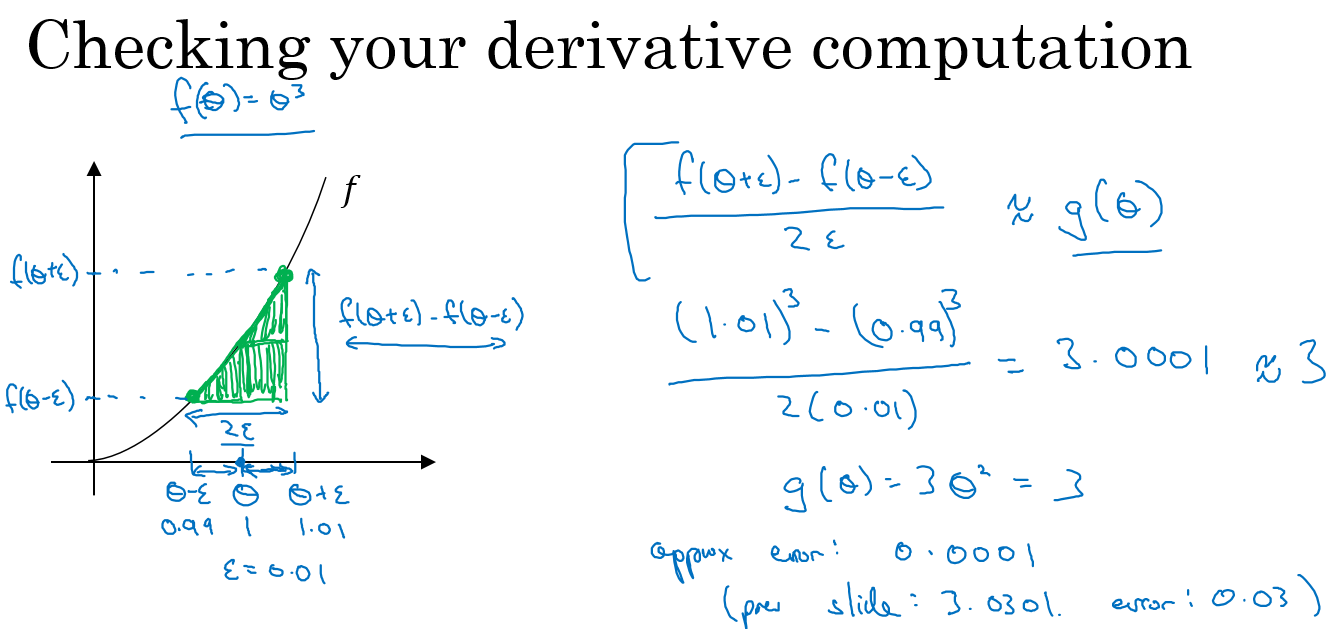• 双边导数：
f(θ)=limε0=f(θ+ε)(θε)2ε

误差：O(ε2)$O(\varepsilon^{2})$
• 单边导数：
f(θ)=limε0=f(θ+ε)(θ)ε

误差：O(ε)$O(\varepsilon)$

### 13. 梯度检验

#### 连接参数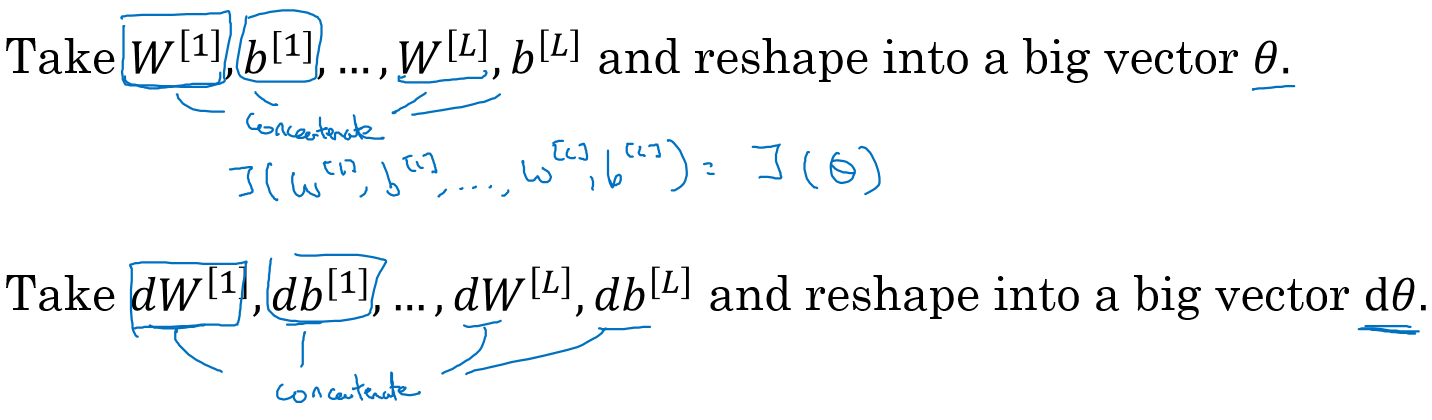#### 进行梯度检验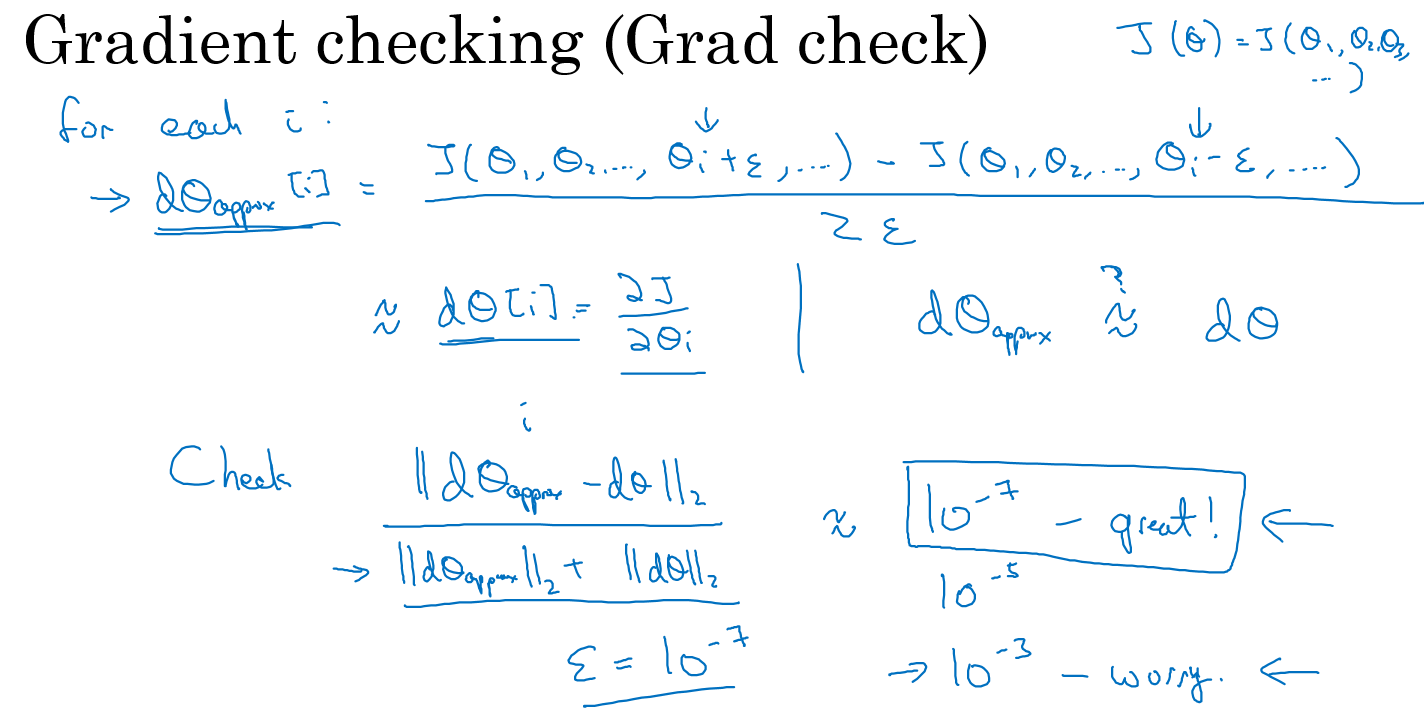||dθapproxdθ||2||dθapprox||2+||dθ||2

### 14. 实现梯度检验 Notes

• 不要在训练过程中使用梯度检验，只在debug的时候使用，使用完毕关闭梯度检验的功能；
• 如果算法的梯度检验出现了错误，要检查每一项，找出错误，也就是说要找出哪个dθapprox[i]$d\theta_{approx}[i]$dθ$d\theta$的值相差比较大；
• 不要忘记了正则化项；
• 梯度检验不能与dropout同时使用。因为每次迭代的过程中，dropout会随机消除隐层单元的不同神经元，这时是难以计算dropout在梯度下降上的代价函数J；
• 在随机初始化的时候运行梯度检验，或许在训练几次后再进行。（没听懂大师这个点的意思？）